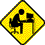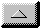Publications Department

Zone Plate (reflecting) Fresnel Antennas
for Amateur SETI -- Part 1

by Henry Wallace (ultrasonic@worldnet.att.net)

There has been much discussion on the SETI email list of Zone Plate (reflecting) Fresnel, or "bullseye," antennas. A "bullseye" is American slang for a target used in archery and shooting practice which consists of concentric red rings surrounding a central red disc. The Zone Plate antenna is just such a pattern, but the red is replaced by metallic reflectors, and the area between the red is replaced by nonreflectors. The entire antenna is flat, that is, all reflecting and nonreflecting "zones" are intended to be confined to the x,y plane.

The current design calls for a central disc reflector surrounded by seven concentric reflecting zones. The following BASIC program allows one to predict the locations of the borders of the zones:

```10 REM Fresnel Zone Plate Reflecting Bullseye 20 R0= 12.92 : F0= 1421E6 : C= 3E8 : LA= C/F0 : N=1 30 FOR S=0 TO R0 STEP R0/10000 40 R=sqr( R0*R0 + S*S ) 50 IF ( R-R0) < N*LA/2 THEN 70 60 PRINT N,S : N=N+1 70 NEXT S 80 END ```

Here F0 is the frequency (1420 MHz ), LA is the wavelength, R0 is the height above ground to the 45 degree feedhorn (40 feet or 12.92 meters). And S is the distance from the center of the bullseye to the border, in meters.

When RUN, this program produces about 56 borders, or about 23 coincentric bands, with alternating bands reflecting or absorbing. Wayne S. (or Aleph Null on SETI net) suggested placing such a reflecting array 1/4 wavelength (about two inches) above a reflecting groundplane. This will probably work, but I have yet to fully simulate it.

The radius of the central disc is 1.695 meters. The seven rings are best described by their "starting" and "ending" radii, and are (in meters):

1 2.402 2.947
2 3.409 3.820
3 4.192 4.537
4 4.859 5.163
5 5.453 5.729
6 5.995 6.252
7 6.500 6.740

Up the z axis 13.55 meters is a feedhorn looking down at the bullseye. The feedhorn has a 45 degree reception angle, to receive energy from all the zones, including the seventh zone. The feedhorn is located directly above the center of the central disc reflector.

Actually, this is only the most rudimentary beginning of the simulation which sums the contributions from all these zones at the feedhorn. However, the remainder of the program will just add up the contributions from alternate zones, using Cos(Kr), Sin(kr) terms (K is just wave-number K=2*pi/ LA) and the area of each contribution (da=2*pi*s*ds). Once the Cos terms are all added up, and the Sin terms are added up, the net amplitude is a=sqr(Cos^2+Sin^2), which is the "zero order" straight-up amplitude.

The off-axis terms just add a linear phase- term in X (or Y), if the bullseye is in the X,Y plane.

Here is the first Zone Plate antenna simulation:

```10 REM Fresnel Zone Plate Antenna 20 DIM S(100) 30 Pi=3.14159 40 R0= 13.55: F0=1421E6: C= 3E8 : LA= C/F0 : N=1 50 FOR S=0 TO R0/2 STEP R0/10000 60 R= SQR(R0*R0+S*S) 70 IF (R-R0)< N*LA/2 THEN 100 80 PRINT N,S 90 S(N)= S : N=N+1 100 NEXT S: S(0)=LA 105 F1=1420E6 :LA= C/F1 : REM F1=OPERATING FREQ. 110 K= 2*PI/LA 120 FOR DG=0 TO 100 STEP .1 : TH=DG/57.325: U3=SIN(TH) 130 N=1: CT=0 : ST=0 : GOSUB 1000 140 FOR N=3 TO 100 STEP 2 : PRINT N;" "; 150 IF S(N)=0 THEN 180 160 GOSUB 1000 170 NEXT N 180 A= SQR( CT*CT+ST*ST) 190 PRINT DG, A 200 NEXT DG 210 END 1000 REM Do a Band 1010 FOR RD= S(N-1) TO S(N) STEP LA/100 1020 R= SQR( R0*R0+RD*RD) : P7=K*(R-R0) 1030 TS= LA / (RD*100) 1040 FOR AD=0 TO Pi STEP TS 1050 X=RD*COS(AD) : PH= K*X*U3 1060 CT= CT+COS(P7-PH) : ST=ST+SIN(P7-PH) 1070 NEXT AD 1080 NEXT RD 1090 RETURN```

The groundplane is NOT included in this simulation. All dimensions are in meters, angles in radians, except for DG which is the off-axis pointer, and is in degrees.

R0 is feedhorn height above the bullseye, and is also approximate bullseye outer diameter. LA is wavelength. A is the computed amplitude of the received signal. It is expressed as linear: to "normalize" the beampattern response, each off-axis A should be divided by the A for zero degrees, and 20*log of this term be taken.

Lines 10-100 set up the "borders" between the Zones, and load these radii into the array S[N], and prints out these borders with their corresponding index N.

Lines 1000-1090 (the "Do a Band" subroutine) does most of the work. The most nested loop rotates the angle AD ( for Angle in Disc ) in steps of size determined by line 1030, which is set at a step size of 1/100 wavelength. Rotation of AD through Pi radians is sufficient, as continued rotation only duplicates. The outer loop in "Do a Band" repeats the process for all eight active zones of this design, skipping those zones from which energy reaching the feedhorn is out-of-phase. Line 1010 sets the step size in radius at 1/100 wavelength.

This program was written and debugged on an Apple II computer in Apple Basic. The variables used in debugging were for a 10 foot diameter antenna with only ONE zone surrounding the central disc, and step sizes were 1/10 wavelength . This was done so that this program could be debugged and presented to the Group within a reasonable time. The Apple II is REALLY SLOW. We suspect ET will come before the Apple II finished the simulation above.

O.K., you Computer Masters: rewrite this thing in "C" and use the math coprocessors for the Sin, Cos. The simulation is accurate, and produces the expected tight beams and sidelobes. There may be a typo left, but I don't think so.

While I have been working on the Zone Plate simulation, I have been aware of some of the discussions about gratings/etc.

I AM CONCERNED that the simulated disc may not perform as well as the simulation predicts. If it were constructed with a "specular" metallic surface, composed of hills/valleys on the order of 1/4 wave high, I would expect it to act nearly as good as the simulations predict. But with entirely "flat" construction techniques, I am not entirely sure. Currently we are considering perfect, flat metallic reflectors. BUT WE WOULD be most interested in simulation results with imperfect, or more "specular" metallic surfaces, like an "imperfect" surface finish.

I had originally thought that, because the thinner zones were further removed from the feedhorn, they would "scatter" the incident RF enough so that a large portion would enter the feedhorn. The outer zone is only 1.1 wavelength wide, and such a narrow metallic surface might scatter the energy sufficiently.

Several approaches suggest themselves if it is determined that the "specularity" of these surfaces needs to be increased. I have seen the suggestions of a sinusoidal surface, and noted the suggestions of "tilted" surfaces. In optics, this is called "blazing". An approach might be to divide each of these zones into many smaller ( thinner, say 1 cm wide ) "minizones". There was work done in the 1950's using foam plastics as lenses: it was found that the increased dielectric constant of certain foams could be used to produce what today we would call a "phase grating".

Whatever solution works best will ultimately be determined by experimentation. And not necessarily on large systems. Many good graduate-level EE labs have HP microwave benches capable of measuring the scattering angles of 21 cm radiation from test surfaces. But as with all scientific progress, experimentation will be the key.email theWebmaster | Home | General | Memb Svcs | Publications | Press | Technical | Internet | Index | entire website copyright © The SETI League, Inc. this page last updated 28 December 2002Top of Page